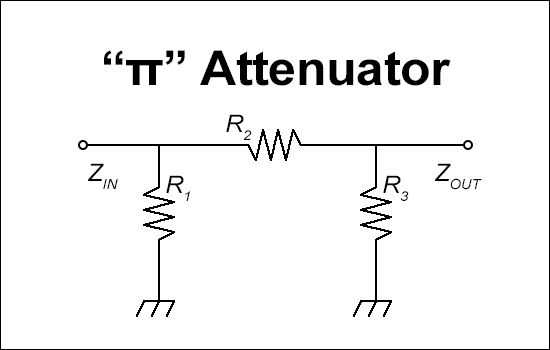# Pi Attenuator Calculator

This calculator calculates the resistor values of the attenuator from the input impedance(Zin), output impedance(Zout), and attenuation.

If the input impedance is greather than the output impedance(Zin>Zout), the level of attenuation must be at least √(Zin/Zout)+√((Zin/Zout)-1)[dB].

If the input impedance is less than the output impedance(Zin<Zout), the level of attenuation must be at least √(Zout/Zin)+√((Zout/Zin)-1)[dB].

Output values are rounded to the fourth decimal place.### Input

Attenuation:
dB

Input Impedance (Zin):

Output Impedance (Zout):